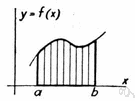# integral

(redirected from Area under a curve)
Also found in: Thesaurus, Medical, Encyclopedia.

## in·te·gral

(ĭn′tĭ-grəl, ĭn-tĕg′rəl)
1. Essential or necessary for completeness; constituent: The kitchen is an integral part of a house.
2. Possessing everything essential; entire.
3. (ĭn′tĭ-grəl) Mathematics
a. Expressed or expressible as or in terms of integers.
b. Expressed as or involving integrals.
n.
1. A complete unit; a whole.
2. (ĭn′tĭ-grəl) Mathematics
a. A number computed by a limiting process in which the domain of a function, often an interval or planar region, is divided into arbitrarily small units, the value of the function at a point in each unit is multiplied by the linear or areal measurement of that unit, and all such products are summed.
b. A definite integral.
c. An indefinite integral.

[Middle English, from Old French, from Medieval Latin integrālis, making up a whole, from Latin integer, complete; see integer.]

in′te·gral′i·ty (-grăl′ĭ-tē) n.

## integral

1. (often foll by to) being an essential part (of); intrinsic (to)
2. intact; entire
3. formed of constituent parts; united
4. (Mathematics) maths
a. of or involving an integral
b. involving or being an integer
n
5. (Mathematics) maths the limit of an increasingly large number of increasingly smaller quantities, related to the function that is being integrated (the integrand). The independent variables may be confined within certain limits (definite integral) or in the absence of limits (indefinite integral). Symbol:
6. a complete thing; whole
integrality n

## in•te•gral

(ˈɪn tɪ grəl, ɪnˈtɛg rəl)

1. of or belonging as an essential part of the whole; necessary to completeness; constituent: an integral part.
2. composed of parts that together constitute a whole.
3. entire; complete; whole.
4. pertaining to or being an integer; not fractional.
5. pertaining to or involving mathematical integrals.
n.
6. an integral whole.
7. Math.
a. Also called Riemann integral. the numerical measure of the area bounded above by the graph of a given function, below by the x-axis, and on the sides by ordinates drawn at the endpoints of a specified interval.
b. a primitive.
c. any of several analogous quantities.
[1545–55; < Medieval Latin integrālis. See integer, -al1]
in`te•gral′i•ty, n.

## in·te·gral

(ĭn′tĭ-grəl)
1. Involving or expressed as an integer or integers.
2. In calculus, the result of integration.
ThesaurusAntonymsRelated WordsSynonymsLegend:
 Noun 1integral - the result of a mathematical integration; F(x) is the integral of f(x) if dF/dx = f(x)figuring, reckoning, calculation, computation - problem solving that involves numbers or quantitiesindefinite integral - the set of functions F(x) + C, where C is any real number, such that F(x) is the integral of f(x)definite integral - the integral of a function over a definite interval Adj. 1 integral - existing as an essential constituent or characteristic; "the Ptolemaic system with its built-in concept of periodicity"; "a constitutional inability to tell the truth"intrinsic, intrinsical - belonging to a thing by its very nature; "form was treated as something intrinsic, as the very essence of the thing"- John Dewey 2 integral - constituting the undiminished entirety; lacking nothing essential especially not damaged; "a local motion keepeth bodies integral"- Bacon; "was able to keep the collection entire during his lifetime"; "fought to keep the union intact"whole - including all components without exception; being one unit or constituting the full amount or extent or duration; complete; "gave his whole attention"; "a whole wardrobe for the tropics"; "the whole hog"; "a whole week"; "the baby cried the whole trip home"; "a whole loaf of bread" 3 integral - of or denoted by an integer

## integral

1. Rituals form an integral part of any human society.
essential
2. This is meant to be an integral service.
whole

## integral

1. Constituting or forming part of the essence of something:
2. Lacking nothing essential or normal:
noun
An organized array of individual elements and parts forming and working as a unit:
Translations
integrál
integraali
integral
integrál
heilduntegrun
integral

## integral

[ˈɪntɪgrəl]
A. ADJ (= essential) [part] →
it is an integral part of the plan
B. N (Math) →
C. CPD integral calculus N (Math) →

## integral

an integral part of sth → une partie intégrante de qch
to be an integral part of sth, to form an integral part of sth → faire partie intégrante de qch
an integral element of sth → un élément constitutif de qch, un élément à part entière de qch
an integral component of sth → un composant à part entière de qch
to play an integral role in sth → jouer un rôle à part entière dans qch
to be integral to sth → faire partie intégrante de qchintegral calculus n